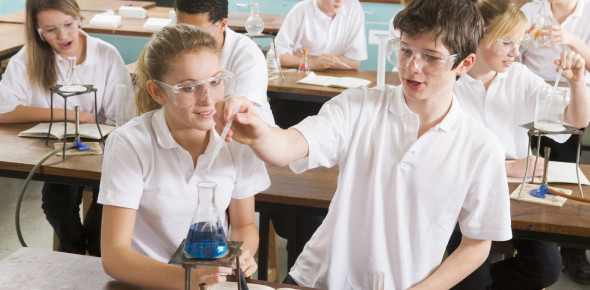# Science Quiz For Class 9

14 Questions | Total Attempts: 67160SettingsIn this class, we covered matters and what it entails. The Science quiz for class 9 below includes five of the topics we have covered so far. The only way you can answer these questions with ease is if you were attentive. Give them a try and see how high you score. Good luck!

• 1.
Distance is a ________________ quantity.
• A.

Scaler

• B.

Vector

• C.

Can't say

• 2.
Displacement is a ________________ quantity.
• A.

Vector

• B.

Scaler

• 3.
Speed is a ________________ quantity.
• A.

Vector

• B.

Scaler

• 4.
Displacement = 0
• A.

True

• B.

False

• 5.
If a body covers a full circle, then the displacement is:
• A.

1

• B.

0

• C.

Distance

• D.

Not sufficient data

• 6.
Ice is:
• A.

Solid

• B.

Liquid

• C.

Gas

• D.

Plasma

• 7.
Plasma consist _________ particles.
• A.

Energetic/exited

• B.

Dull

• C.

Tightly packed

• 8.
What is latent heat of fusion of ice:
• 9.
ER help in Detoxification:
• A.

True

• B.

False

• 10.
Golgi apparatus helps in the cell division.
• A.

True

• B.

False

• 11.
Ribosomes make __________.
• A.

Lipids

• B.

Fats

• C.

Proteins

• D.

Starch

• 12.
SER involves Storage.
• A.

True

• B.

False

Related TopicsBack to top